﻿ ScatterPlot – Algosim documentation
Algosim documentation: ScatterPlot

# ScatterPlot

Creates an XY or XYZ scatter plot.

## Syntax

• `ScatterPlot(data)`

• `data` is a sequence of (X, Y) points

• `ScatterPlot(data)`

• `data` is a sequence of (X, Y, Z) points

• `ScatterPlot(data)`

• `data` is a sequence of (X, Y, Z, colour, radius) points

## Description

### 2D points

If `data` is a sequence of (X, Y) points, then `ScatterPlot(data)` creates a scatter plot from this data.

Technically, `data` can be a plain sequence of points as function arguments, a list of points, or an n×2 real matrix in which each row represents a single point.

The chart is shown in the current diagram and a reference to the chart is returned.

To plot the graph of a function using points only, you may combine `ScatterPlot` with the `graph` function. But it is more convenient to use `plot` instead.

### 3D points

Similarly, if `data` is a sequence of (X, Y, Z) points, then `ScatterPlot(data)` creates a scatter plot from this data.

Technically, `data` can be a plain sequence of points as function arguments, a list of points, or an n×3 real matrix in which each row represents a single point.

The chart is shown in the current scene and a reference to the chart is returned.

To plot the graph of a function using points only, you may combine `ScatterPlot` with the `graph` function. But it is more convenient to use `plot` instead.

Additionally, you can plot a set of points in which each point has its own colour and radius. This set needs to be a set of (X, Y, Z, colour, radius) points, and can be specified in any of the ways described above for (X, Y, Z) point sets.

## Examples

### 2D points

`ScatterPlot(❨1, 1❩, ❨2, 5❩, ❨3, −2❩)`

or

`ScatterPlot('(❨1, 1❩, ❨2, 5❩, ❨3, −2❩))``A ≔ ❨❨1, 1❩, ❨2, 5❩, ❨3, −2❩❩`
```⎛ 1   1⎞
⎜ 2   5⎟
⎝ 3  −2⎠
```
`ScatterPlot(A)``ScatterPlot(graph(sin, −2⋅π, 2⋅π))`### 3D points

`ScatterPlot(10⋅RandomMatrix(1000, 3))`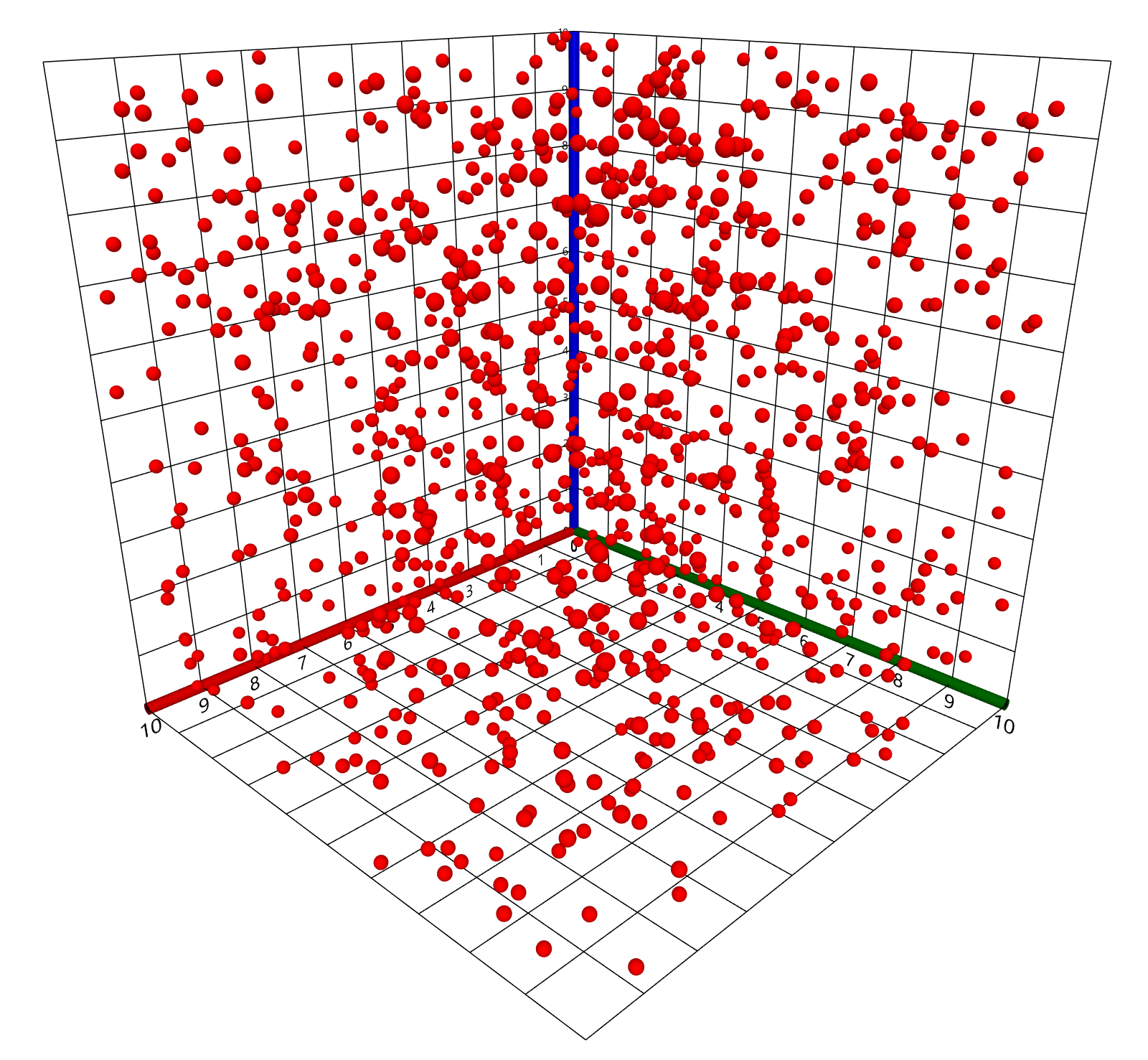`ScatterPlot(graph((x, y) ↦ sin(√(x^2 + y^2)), ❨−10, 10❩, ❨−10, 10❩))`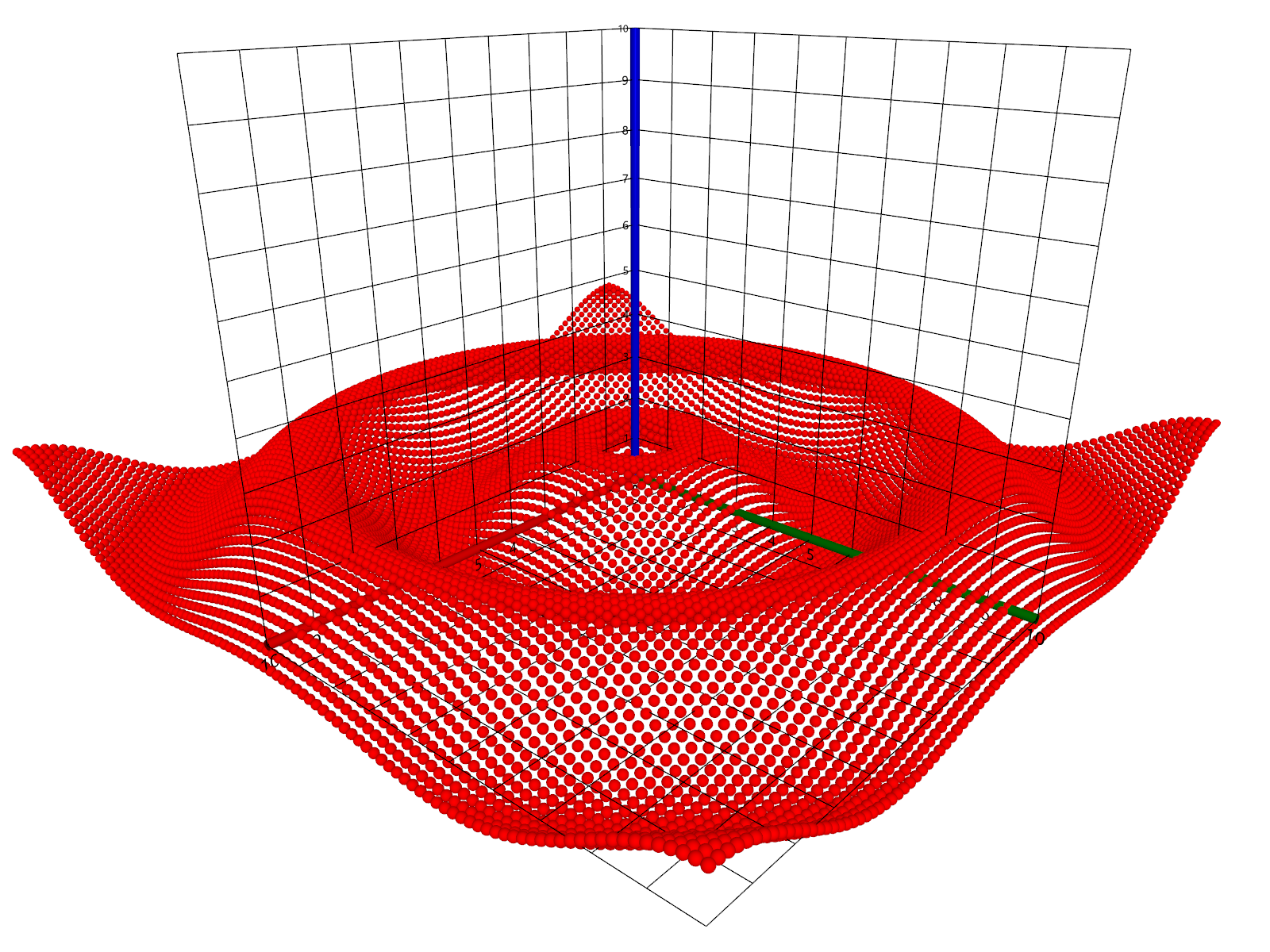`F ≔ (x, y, z) ↦ ❨x, y, z, integer(rgb(x / 10, y / 10, z / 10)), 5.0❩;`
`ScatterPlot([0, 10, 1]^3 @ F)`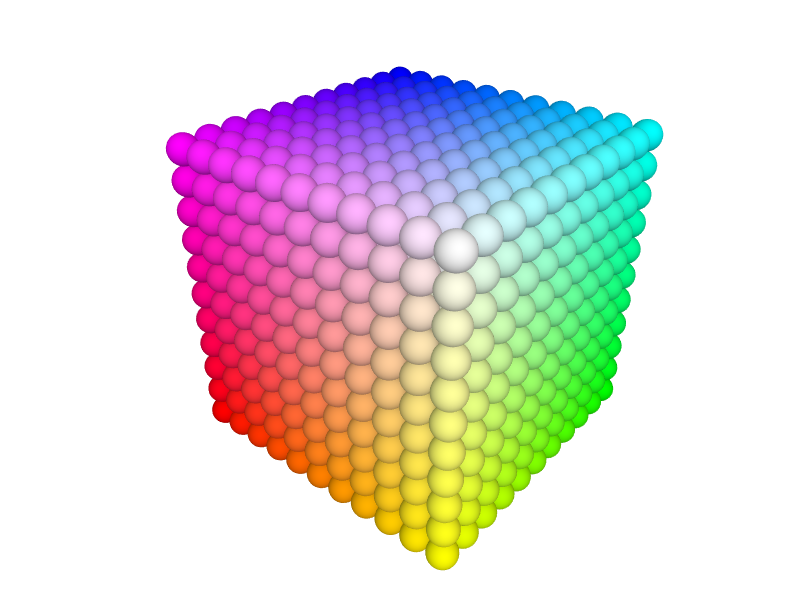`F ≔ (x, y, z) ↦ ❨x ⋅ RandomReal(), y ⋅ RandomReal(), z ⋅ RandomReal(), integer(rgb(x / 10, y / 10, z / 10)), RandomReal(5.0)❩;`
`ScatterPlot([0, 10, 0.5]^3 @ F)`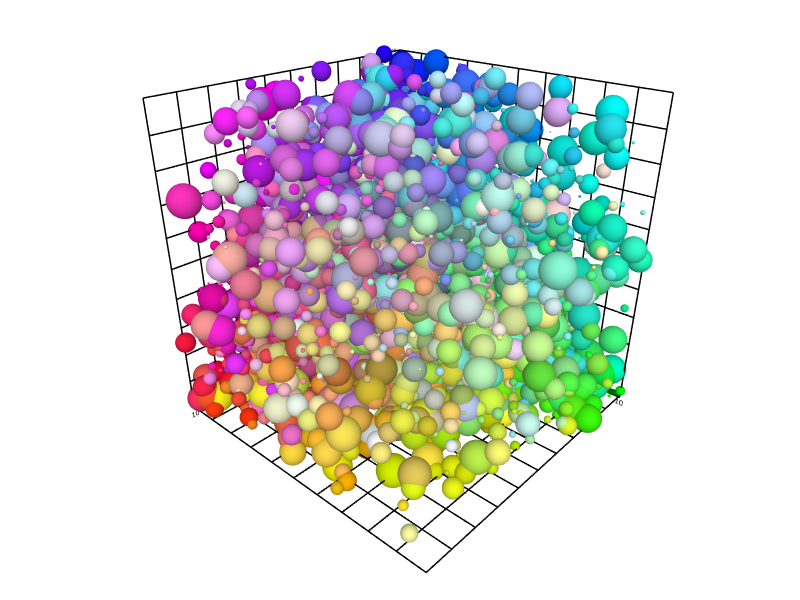`F ≔ (θ, φ) ↦ ❨10⋅sin(θ)⋅cos(φ), 10⋅sin(θ)⋅sin(φ), 10⋅cos(θ), integer(hsv(180⋅(θ+2⋅φ)/π, 1.0, 1.0)), 5.0❩;`
`ScatterPlot([0, π, π/50] × [0, 2⋅π, π/50] @ F)`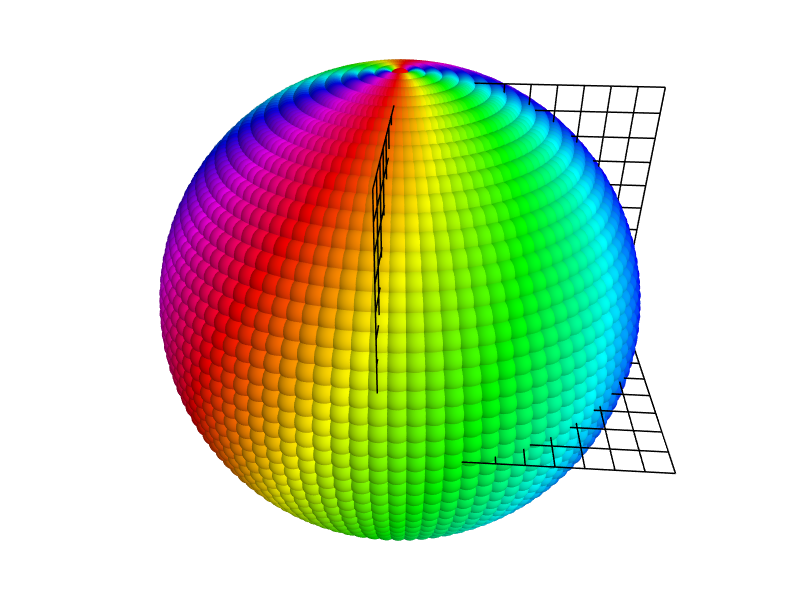The above discussion details how to plot a sequence of points in ℝ² or ℝ³. But what if you have a list (or vector) containing real numbers, that is, elements of ℝ? Like this:

```3.1, 2.9, 3.5, 3.2, 3.4, 3.0, 2.9, 3.7, 3.2, 3.5
```

One way to visualise such a sequence is to transform it into a sequence of points in ℝ²:

`(1, 3.1), (2, 2.9), (3, 3.5), (4, 3.2), (5, 3.4), (6, 3.0), (7, 2.9), (8, 3.7), (9, 3.2), (10, 3.5)`

Then this sequence can be plotted as before. To accomplish this, one can use the SequenceVector function along with MatFromCols:

```D ≔ ❨3.1, 2.9, 3.5, 3.2, 3.4, 3.0, 2.9, 3.7, 3.2, 3.5❩;
plot(MatFromCols(SequenceVector(#D), D))
```See the example in LineSegment for a different approach, in which a one-dimensional diagram is used to visualise a monotonic sequence.